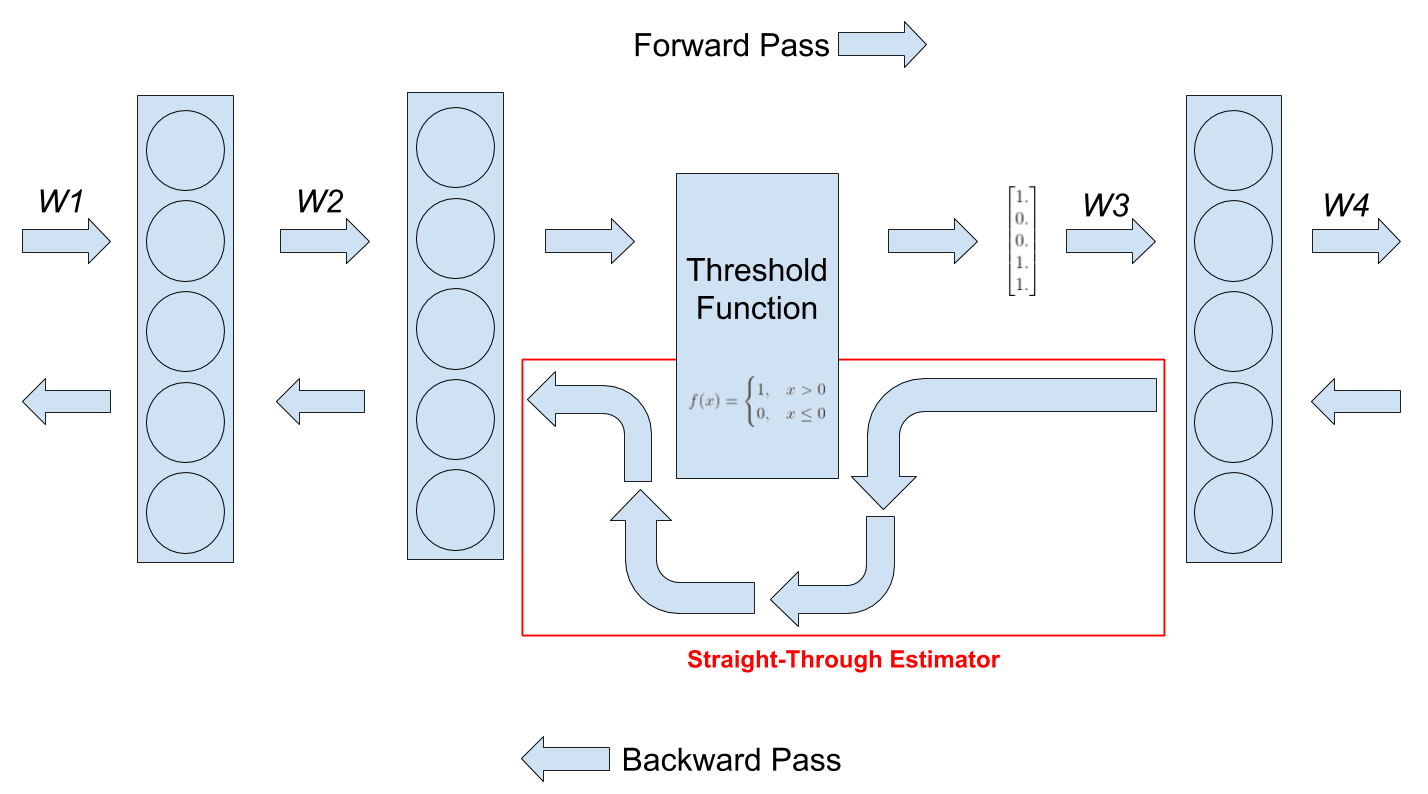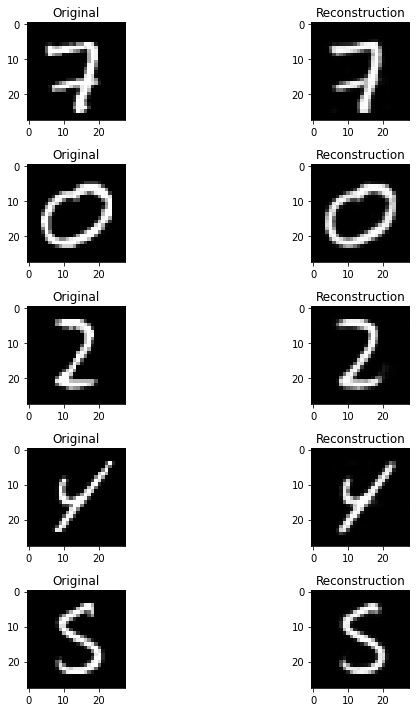Sometimes we want to put a threshold function at the output of a layer. This can be for a variety of reasons. One of them is that we want to summarize the activations into binary values. This binarization of activations can be useful in autoencoders.

However, thresholding poses a problem during backpropagation. The derivative of threshold functions is zero. This lack of gradient results in our network not learning anything. To solve this problem we use straight-through estimators (STE).

# What is a Straight-Through Estimator?

Lets suppose we want to binarize the activations of a layer using the following function:

$f(x) = \begin{cases} 1, & x > 0 \\ 0, & x \le 0 \end{cases}$

This function will return 1 for every value that is greater than 0 otherwise it will return 0.

As mentioned earlier, the problem with this function is that its gradient is zero. To overcome this issue we will use a straight-through estimator in the backward pass.

A straight-through estimator is exactly what it sounds like. It estimates the gradients of a function. Specifically it ignores the derivative of the threshold function and passes on the incoming gradient as if the function was an identity function. The following diagram will help explain it better.You can see how the threshold function is bypassed in the backward pass. That’s it, this is what a straight-through estimator does. It makes the gradient of the threshold function look like the gradient of the identity function.

# Implementation in PyTorch

As of right now, PyTorch doesn’t include an implementation of an STE in its APIs. So, we will have to implement it ourselves. To do this we will need to create a Function class and a Module class. The Function class will contain the forward and backward functionality of the STE. The Module class is where the STE Function object will be created and used. We will use the STE Module in our neural networks.

Below is the implementation of the STE Function class:

class STEFunction(torch.autograd.Function):
@staticmethod
def forward(ctx, input):
return (input > 0).float()

@staticmethod


PyTorch lets us define custom autograd functions with forward and backward functionality. Here we have defined an autograd function for a straight-through estimator. In the forward pass we want to convert all the values in the input tensor from floating point to binary. In the backward pass we want to pass the incoming gradients without modifying them. This is to mimic the identity function. Although, here we are performing the F.hardtanh operation on the incoming gradients. This operation will clamp the gradient between -1 and 1. We are doing this so that the gradients do not get too big.

Now, lets implement the STE Module class:

class StraightThroughEstimator(nn.Module):
def __init__(self):
super(StraightThroughEstimator, self).__init__()

def forward(self, x):
x = STEFunction.apply(x)
return x


You can see that we have used the STE Function class we defined in the forward function. To use autograd functions we have to pass the input to the apply method. Now, we can use this module in our neural networks.

A common way to use STE is inside the bottleneck layer of autoencoders. Here is an implementation of such an autoencoder:

class Autoencoder(nn.Module):
def __init__(self):
super(Autoencoder, self).__init__()

self.encoder = nn.Sequential(
nn.Conv2d(1, 64, kernel_size=(4, 4), stride=(2, 2), padding=(1, 1)),
nn.ReLU(),

nn.Conv2d(64, 128, kernel_size=(4, 4), stride=(2, 2), padding=(1, 1)),
nn.BatchNorm2d(128),
nn.ReLU(),

nn.Conv2d(128, 256, kernel_size=(4, 4), stride=(2, 2), padding=(1, 1)),
nn.BatchNorm2d(256),
nn.ReLU(),

nn.Conv2d(256, 512, kernel_size=(4, 4), stride=(2, 2), padding=(1, 1)),
nn.BatchNorm2d(512),
nn.ReLU(),

StraightThroughEstimator(),
)
self.decoder = nn.Sequential(
nn.ConvTranspose2d(512, 256, kernel_size=(5, 5), stride=(2, 2), padding=(1, 1)),
nn.BatchNorm2d(256),
nn.ReLU(),

nn.ConvTranspose2d(256, 128, kernel_size=(5, 5), stride=(2, 2), padding=(1, 1)),
nn.BatchNorm2d(128),
nn.ReLU(),

nn.ConvTranspose2d(128, 64, kernel_size=(4, 4), stride=(2, 2), padding=(1, 1)),
nn.BatchNorm2d(64),
nn.ReLU(),

nn.ConvTranspose2d(64, 1, kernel_size=(4, 4), stride=(2, 2), padding=(1, 1)),
nn.Tanh(),
)

def forward(self, x, encode=False, decode=False):
if encode:
x = self.encoder(x)
elif decode:
x = self.decoder(x)
else:
encoding = self.encoder(x)
x = self.decoder(encoding)
return x


This autoencoder is made for the MNIST dataset. It will compress the 28x28 image into a 1x1 image with 512 channels. Then decode it back to 28x28 image.

I’ve placed the STE at the end of the encoder. It will convert all of the values of the tensor it receives to binary. You might have noticed I’ve used an unconventional forward function. I’ve added two new arguments encode and decode which are either True or False. If encode is set to True, the network will return the output of the encoder. Similarly if decode is set to True, the network expects a valid encoding and it will decode it back to an image.

I trained the autoencoder for 5 epochs on the MNIST dataset with MSE loss. Here are the reconstructions on the test set:As you can see, the reconstructions are pretty good. STEs can be used in neural networks without much loss in performance.

# Full Code

import torch
import torch.nn as nn
import torch.nn.functional as F
import torch.optim as optim
from torchvision import datasets, transforms
import numpy as np
import matplotlib.pyplot as plt
from tqdm import tqdm

device = 'cuda:0' if torch.cuda.is_available() else 'cpu'

# dataset preparation

transform = transforms.Compose([
transforms.ToTensor(),
transforms.Normalize((0.5, ), (0.5, ))
])

# defining networks

@staticmethod
def forward(ctx, input):
return (input > 0).float()

@staticmethod

class StraightThroughEstimator(nn.Module):
def __init__(self):
super(StraightThroughEstimator, self).__init__()

def forward(self, x):
x = STEFunction.apply(x)
return x

class Autoencoder(nn.Module):
def __init__(self):
super(Autoencoder, self).__init__()

self.encoder = nn.Sequential(
nn.Conv2d(1, 64, kernel_size=(4, 4), stride=(2, 2), padding=(1, 1)),
nn.ReLU(),

nn.Conv2d(64, 128, kernel_size=(4, 4), stride=(2, 2), padding=(1, 1)),
nn.BatchNorm2d(128),
nn.ReLU(),

nn.Conv2d(128, 256, kernel_size=(4, 4), stride=(2, 2), padding=(1, 1)),
nn.BatchNorm2d(256),
nn.ReLU(),

nn.Conv2d(256, 512, kernel_size=(4, 4), stride=(2, 2), padding=(1, 1)),
nn.BatchNorm2d(512),
nn.ReLU(),

StraightThroughEstimator(),
)
self.decoder = nn.Sequential(
nn.ConvTranspose2d(512, 256, kernel_size=(5, 5), stride=(2, 2), padding=(1, 1)),
nn.BatchNorm2d(256),
nn.ReLU(),

nn.ConvTranspose2d(256, 128, kernel_size=(5, 5), stride=(2, 2), padding=(1, 1)),
nn.BatchNorm2d(128),
nn.ReLU(),

nn.ConvTranspose2d(128, 64, kernel_size=(4, 4), stride=(2, 2), padding=(1, 1)),
nn.BatchNorm2d(64),
nn.ReLU(),

nn.ConvTranspose2d(64, 1, kernel_size=(4, 4), stride=(2, 2), padding=(1, 1)),
nn.Tanh(),
)

def forward(self, x, encode=False, decode=False):
if encode:
x = self.encoder(x)
elif decode:
x = self.decoder(x)
else:
encoding = self.encoder(x)
x = self.decoder(encoding)
return x

net = Autoencoder().to(device)
optimizer = optim.Adam(net.parameters(), lr=0.001, betas=(0.5, 0.999))
criterion_MSE = nn.MSELoss().to(device)

# train loop

epoch = 5
for e in range(epoch):
print(f'Starting epoch {e} of {epoch}')
for X, y in tqdm(trainloader):
X = X.to(device)
reconstruction = net(X)
loss = criterion_MSE(reconstruction, X)
loss.backward()
optimizer.step()
print(f'Loss: {loss.item()}')

# test loop

i = 1
fig = plt.figure(figsize=(10, 10))

for X, y in testloader:
X_in = X.to(device)
recon = net(X_in).detach().cpu().numpy()

if i >= 10:
break